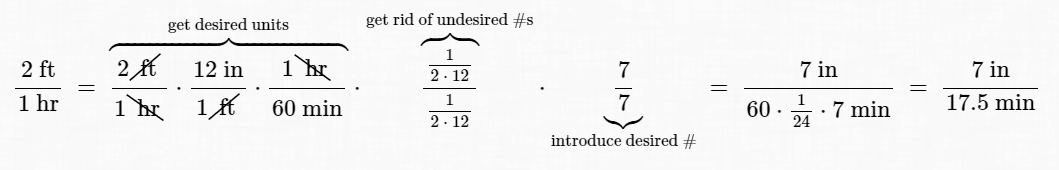#Rate Problems

The Unit Conversion Tables should be helpful for this section. (The link will open in a new window, so you can keep this information close by.)

DEFINITION rate
A rate is a comparison of two quantities that are measured in different units.

Here are some examples of rates:

$\displaystyle\frac{\$5}{\text{hr}}$, also commonly seen as$\,\$5/\text{hr}\,,$ and read as ‘five dollars per hour’

$\displaystyle\frac{40\text{ miles}}{3\text{ days}}$,   read as ‘forty miles per three days’

$\displaystyle\frac{10\text{ kg}}{{\text{ in}}^3}$,   read as ‘ten kilograms per cubic inch’

A rate is a mathematical expression, and like all types of mathematical expressions, rates have lots of different names. For example,

$$\cssId{s20}{\frac{\5}{\text{hr}}} \cssId{s21}{= \frac{\10}{2\text{ hr}}} \cssId{s22}{= \frac{\2.50}{30\text{ min}}} \cssId{s23}{= \frac{50\text{ cents}}{6\text{ min}}}$$

Notice that each of these names has a unit of currency (a money unit) in the numerator, and a unit of time in the denominator.

A rate can be renamed to a new desired name, providing the type of units in the numerator and denominator (e.g., length, time, volume, mass/weight) remain the same.

For example, suppose a rate has a unit of length in the numerator, and a unit of volume in the denominator. Then, it can only be renamed to rates that have length in the numerator and volume in the denominator.

Which name should you use? It depends! If you're earning \$5 per hour, and want to know how much you'll earn in$\,30\,$minutes, then$\displaystyle\,\frac{\$2.50}{30\text{ min}}\,$ is a good name. You can get whatever name you need just by multiplying by one, as you'll see below!

Try not to be intimidated by the ‘fractions within fractions’. If you give this method a chance, you'll be solving an incredible variety of problems quickly and efficiently.

Two versions of the solution are given: a one-sentence solution and a two-sentence solution. Both solutions have the same beginning stepsto make sure you know what you're starting with, and what you want to end up with!

## Example

Question:
A snail crawls about $\,2\,$ feet in one hour. How many minutes will it take to crawl $\,7\,$ inches?

## Both Versions of the Solution Start Off the Same Way

• Step 1:   Identify the original rate.
Answer:   $\displaystyle\frac{2\text{ ft}}{1\text{ hr}}$
• Step 2:   Identify the name for the rate that you want. Use $\,x\,$ for any number that is unknown.
Answer:   $\displaystyle\frac{7\text{ in}}{x\text{ min}}$
• Step 3:   Check that the original rate and its desired new name are ‘compatible’; i.e., same types of units in both numerator and denominator.

Answer: Both rates have units of length upstairs (feet and inches), and units of time downstairs (hours and minutes). They're compatible!
Solution #1(one-sentence solution):

This one-sentence solution is ultra-fast and efficient, once you get used to working with fractions within fractions.

First, you multiply by $\,1\,$ in appropriate forms to get the units you want. Then, you get rid of the numbers you don't want. Then, you introduce the number you do want! All by just multiplying by $\,1\,$!

If you're not seeing what's going on here, there are lots more details in the text (starting on page 172).

\begin{align} \cssId{s73}{\frac{2\text{ ft}}{1\text{ hr}}} &\cssId{s74}{\ =\ \overbrace{\frac{2\cancel{\text{ ft}}}{1\bcancel{\text{ hr}}} \cdot \frac{12\text{ in}}{1\cancel{\text{ ft}}} \cdot \frac{1\bcancel{\text{ hr}}}{\vphantom{\bcancel{\text{ hr}}}60\text{ min}}}^{\text{get desired units}}}\cr\cr &\qquad\cssId{s75}{\cdot} \cssId{s76}{\overbrace{\frac{\frac1{2\,\cdot\, 12}}{\frac1{2\,\cdot\, 12}}}^{\text{get rid of undesired #s}}}\cr\cr &\qquad\cssId{s77}{\cdot} \cssId{s78}{\underbrace{\frac{7}{7}}_{\text{introduce desired #}}}\cr\cr &\cssId{s79}{\ =\ \frac{7\text{ in}}{60\cdot\frac{1}{24}\cdot 7\text{ min}}} \cssId{s80}{\ =\ \frac{7\text{ in}}{17.5\text{ min}}} \end{align}

(Here's an image with it all on one line:)Here it is again, in the most compact form. This is the form you'll use when you get really good at this method!

\begin{align} \cssId{s83}{\frac{2\text{ ft}}{1\text{ hr}}}\ &\cssId{s84}{= \frac{2\text{ ft}}{1\text{ hr}} \cdot \frac{12\text{ in}}{1\text{ ft}} \cdot \frac{1\text{ hr}}{60\text{ min}}} \cssId{s85}{\cdot} \cssId{s86}{\frac{\frac1{24}}{\frac1{24}}} \cssId{s87}{\cdot} \cssId{s88}{\frac{7}{7}}\cr &\cssId{s89}{= \frac{7\text{ in}}{17.5\text{ min}}} \end{align} Thus, it takes $\,17.5\,$ minutes for the snail to crawl $\,7\,$ inches.
Solution #2(two-sentence solution):

Here's the two-sentence solution. The first sentence renames the rate with the desired units. The second sentence sets up a proportion, which is then solved for the unknown number.

First sentence:   Convert the given rate to the desired units.

Answer: \begin{align} \cssId{s99}{\frac{2\text{ ft}}{1\text{ hr}}}\ &\cssId{s100}{= \frac{2\text{ ft}}{1\text{ hr}} \cdot \frac{12\text{ in}}{1\text{ ft}} \cdot \frac{1\text{ hr}}{60\text{ min}}}\cr\cr &\cssId{s101}{= \frac{24\text{ in}}{60\text{ min}}} \end{align}

Second sentence:   Set up a proportion to solve for the unknown number.

Answer: $$\cssId{s105}{\frac{24\text{ in}}{60\text{ min}}} \cssId{s106}{= \frac{7\text{ in}}{x\text{ min}}}$$

Now, solve for $\,x\,.$ Ignore all the units; just work with the numbers.

 $\displaystyle 24x = 420$ (cross-multiply) $\displaystyle x = \frac{420}{24} = 17.5$ (solve for $\,x\,$)

Thus, it takes $\,17.5\,$ minutes for the snail to crawl $\,7\,$ inches.

## Examples

An object travels $\,5\text{ ft}\,$ in $\,8\text{ min}\,.$

Question: How many $\,\text{ft}\,$ will it travel in $\,20\text{ min}\,$?
Answer: $12.5$
Question: How many $\,\text{min}\,$ will it take to travel $\,43\text{ cm}\,$?
Answer: $2.293333$

## Practice

Feel free to use scrap paper and a calculator to compute your answers.

All answers are either exact, or rounded to six decimal places. It is possible to get $\,0.000000\,$ as an answer.